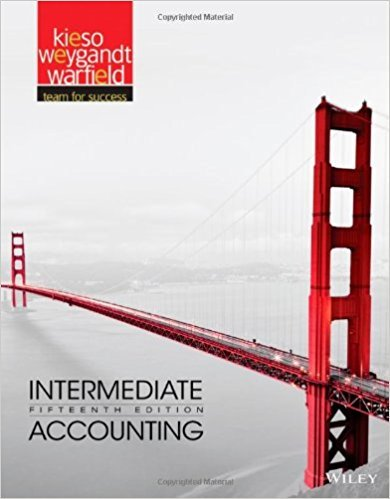×

×

# Solutions for Chapter 14: Long-Term Liabilities## Full solutions for Intermediate Accounting | 15th Edition

ISBN: 9781118147290Solutions for Chapter 14: Long-Term Liabilities

Solutions for Chapter 14
4 5 0 397 Reviews
24
0
##### ISBN: 9781118147290

Summary of Chapter 14: Long-Term Liabilities

This expansive textbook survival guide covers the following chapters and their solutions. Chapter 14: Long-Term Liabilities includes 30 full step-by-step solutions. This textbook survival guide was created for the textbook: Intermediate Accounting, edition: 15. Since 30 problems in chapter 14: Long-Term Liabilities have been answered, more than 39280 students have viewed full step-by-step solutions from this chapter. Intermediate Accounting was written by and is associated to the ISBN: 9781118147290.

Key Business Terms and definitions covered in this textbook
• accounting profit

total revenue minus total explicit cost

• appreciation

an increase in the value of a currency as measured by the amount of foreign currency it can buy

• budget deficit

a shortfall of tax revenue from government spending

• capital requirement

a government regulation specifying a minimum amount of bank capital

• circular-flow diagram

a visual model of the economy that shows how dollars flow through markets among households and firms

• cost–benefit analysis

a study that compares the costs and benefits to society of providing a public good

• cross-price elasticity of demand

a measure of how much the quantity demanded of one good responds to a change in the price of another good, computed as the percentage change in quantity demanded of the first good divided by the percentage change in price of the second good

• cross-price elasticity of demand

a measure of how much the quantity demanded of one good responds to a change in the price of another good, computed as the percentage change in quantity demanded of the first good divided by the percentage change in price of the second good

• depression

a severe recession

• efficiency wages

above-equilibrium wages paid by firms to increase worker productivity

• efficient scale

the quantity of output that minimizes average total cost

• incentive

something that induces a person to act

• inferior good

a good for which, other things being equal, an increase in income leads to a decrease in demand

• life cycle

the regular pattern of income variation over a person’s life

• marginal change

a small incremental adjustment to a plan of action

• median voter theorem

a mathematical result showing that if voters are choosing a point along a line and each voter wants the point closest to his most preferred point, then majority rule will pick the most preferred point of the median voter

• money

the set of assets in an economy that people regularly use to buy goods and services from other peopl

• monopoly

a firm that is the sole seller of a product without close substitutes

• principal

a person for whom another person, called the agent, is performing some act

• theory of liquidity preference

Keynes’s theory that the interest rate adjusts to bring money supply and money demand into balance